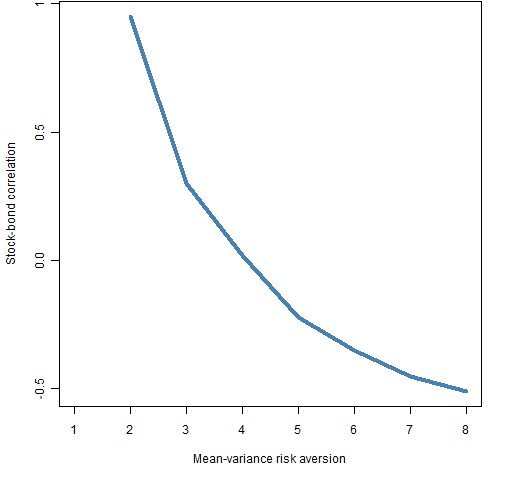Want to share your content on R-bloggers? click here if you have a blog, or here if you don't.

Some thoughts and resources regarding a popular fund management buzzword.

## The idea

Given asset categories (like stocks, bonds and commodities) create a portfolio where each category contributes equally to the portfolio variance.

## Two operations

There are two cases in creating a risk parity portfolio:

• the universe is the asset categories
• the universe is the assets within the categories

In the first case the resulting portfolio is completely defined by the assumed variance matrix.  If we demanded an equal weight portfolio, then how much we had of each asset would be completely determined by the prices of the assets.  The same thing happens with risk parity.

In the second case there is more freedom.  The portfolio variance can vary along with the specific assets that are included and their weights.

## Assumptions

What do we need to believe in order to want a risk parity portfolio?

We might naively think that it has to do with the equality of the utility of the categories.  As we’ll see that is sometimes true, but it depends on our utility.

We’ll use the stock-bond data given in Asness, Frazzini and Pedersen (Table 2, page 22).  This lists the excess return of stocks as 5.96% with volatility of 15.71%, and the excess return of bonds 2.72% with 5.36% volatility.  We also assume the case that the universe is just the two categories: stocks and bonds.

#### mean-variance utility

With this data and assuming that we have a mean-variance utility (portfolio return minus portfolio variance times risk aversion — no 2 involved), we look at what the correlation between stocks and bonds must be in order to get the risk parity portfolio.  Figure 1 shows the results.

Figure 1: Required stock-bond correlation to get risk parity portfolio with mean-variance utility.You can see that if you have a risk aversion of 1 that you can’t get the risk parity portfolio. The required correlation is highly dependent on risk aversion.

#### maximum information ratio utility

If the task is to maximize the information ratio (actually the Sharpe ratio since excess returns are used), then we need to increase the excess return of stocks to 7.97% in order to get the risk parity portfolio — this makes the information ratio of stocks equal to that of bonds.  The correlation between the categories doesn’t matter.

## Thought experiment

Suppose we have a risk parity portfolio of stocks and bonds and then we want to add commodities.  The risk of commodities suddenly goes from zero to one-third.  If we then split bonds into government bonds and corporate bonds, then the total amount of risk in bonds goes from one-third to one-half.

Is this rational?

## In Portfolio Probe

#### universe is categories

This would just take an additional argument in the optimizer:

risk.fraction = 1/Nassets + eps

The eps is needed because of the discreteness of trading. It would be sufficient to make eps the largest unit cost divided by the gross value of the portfolio.

#### universe is assets within categories

The constraint bounds would be set like:

linB[categRows, 2] <- 1/Ncateg + eps

and then optimizer arguments (available as of version 1.04) would be:

lin.bounds=linB, lin.style="varfraction"

## Resources

A lot of documents on risk parity are either written to promote it, or to tear it apart.

### Pro

“Leverage Aversion and Risk Parity” by Clifford Asness, Andrea Frazzini and Lasse Pedersen.

“Risk parity: Case study: ATP” by Henrik Gade Jepsen.

“Counter-Point to Risk Parity Critiques” by Ed Peters.

### Con

“Risk Parity: Nice idea, awkward reality” by Joseph Mariathasan.

“The Hidden Risks of Risk Parity Portfolios” by Ben Inker.

“The Perils of Parity” by Ruban and Melas. Mostly about leverage. Free registration is required.

### General

“Risk Parity 101″ from Hammond Associates.

Special issue of The Journal of Investing on risk parity from Spring 2011.

“Risk Parity: In the spotlight after 50 years” by Christopher Levell.

“Survey Finds Asset Owners ‘Get’ Risk Parity” from Plan Sponsor.

“On the Properties of Equally-Weighted Risk Contributions Portfolios” by Maillard, Roncalli and Teiletche.

“Risk Parity Portfolio vs. Other Asset Allocation Heuristic Portfolios” by Chaves, Hsu, Li and Shakernia.

## My take

A good thing about risk parity is that it emphasizes constraints on risk rather than weights.  Now that we have technology to impose constraints on partitions of the portfolio variance it would be good for weight constraints to largely go extinct.

Some of the good features of risk parity is because it moves toward low volatility investing.

The bad part of risk parity is that — in my opinion — it over-constrains portfolios.  Neither am I especially keen on leveraging to make the overly constrained portfolio have an expected return similar to a less constrained portfolio.

## Questions

What am I missing or have wrong regarding what risk parity means?

What am I missing in the way of resources?

## Appendix R

Some R functions that were used to create the analyses are in risk_parity_objs.R.

For instance finding the stock return for the risk parity with different correlations when maximizing the information ratio was done with:

require(PortfolioProbe)
rhovec2 <- seq(-.1, .3, length=50)
irstockret <- numeric(50)
names(irstockret) <- rhovec2

for(i in 1:50) irstockret[i] <- uniroot(function(x) pp.sbir(rhovec2[i], x), c(0,.5))\$root

Subscribe to the Portfolio Probe blog by Email